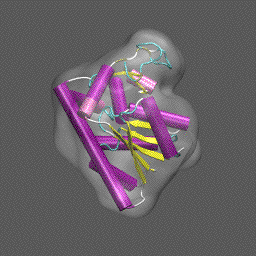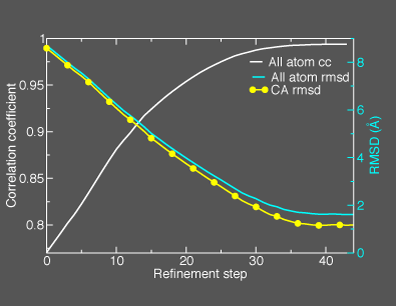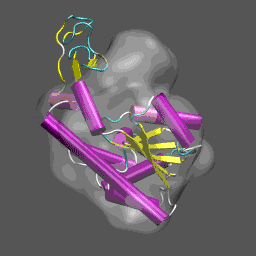Purpose This tutorial is designed to illustrate the application of NMFF and the MMTSB Tool Set to the flexible refinement of an atomic level structure into an electron density map from cryo-EM, electron tomography, etc. In this exercise we will create an electron density map corresponding to the closed structure of adenylate kinase (right figure) and refine the atomic coordinates of the open conformation (right figure) into this density using the normal mode flexible fitting algorithm. To perform the calculations in this tutorial you will need to have the NMFF suite of programs installed. Installation instructions for NMFF can be found here. We will also use parts of the MMTSB Tool Set. Other useful programs include vmd, which is used to visualize the structural and electron density data, however, your favorite structure/density viewing/manipulation program (e.g., O, etc.) can also be used.Preparing the data First we need to get the "raw" pdb files for the closed (1AKE.pdb) and open (4AKE.pdb) states of adenylate kinase. These can be obtained from the pdb by or directly downloading them using the links above. We want to extract the coordinates from chain A for each system and center the coordinates before computing the electron density map. To do this, for our target molecule 1ake, we use the MMTSB Tool convpdb.pl. The following unix command will do this (Note , unix> denotes the unix system prompt and the command follows.) unix>convpdb.pl -center -nohetero -chain A 1AKE.pdb > 1ake_aa_center.pdb With this command we created the new pdb file named 1ake_aa_center.pdb. Let's now make the electron density map using the NMFF suite component program pdb2sim_map. This program requires three arguments and takes an optional fourth. To make the electron density map representing 1ake_aa_center.pdb at approximately 10 Å resolution with a 1 Å grid spacing, use the command. unix>pdb2sim_map 1ake_aa_center.pdb 5 1ake_aa_center.xpl 1
 Note that the electron density map is written in the X-plor format. Also, see that the 10 Å resolution is achieved by setting the 2nd parameter to 5 (desired resolution/2). The computed electron density is shown enveloping the cartoon structure of the closed form of adenylate kinase in the figure on the right. Next we will superpose chain A from the structure 4AKE.pdb onto the backbone of 1ake_aa_center.pdb and then rotate this conformation by 20° around the x-axis to "misalign" the structure to be refined and the density, for illustrative purposes, using a combination of MMTSB Tools and unix pipes. The tools are convpdb.pl and lsqfit.pl. The command is: unix>convpdb.pl -chain A -nohetero 4AKE.pdb | lsqfit.pl -sel ca 1ake_aa_center.pdb | convpdb.pl -rotatex 20 > 4ake_aa_rotx20.pdbWe are now ready to move on to running the refinement. Note that you can skip these steps and directly download the density 1ake_aa_center.xpl and the coordinates 1ake_aa_center.pdb and 4ake_aa_rotx20.pdb.

The control program for running NMFF is the perl script nmffem.pl. This script reads the input parameters that controls the refinement as well as runs the refinement through calls to component programs that are part of the NMFF suite. The input file is called nmff.inp and contains the following lines:

nmff.inp

original pdb = 4ake_aa_rotx20.pdb
target map = 1ake_aa_center.xpl
maximum displacement = 1.0
first mode to use = 1
maximum number of modes = 20
cutoff = 5.5
nmff directory = src/nmff/em
nma directory = src/nma/rtb
resolution = 10.0

In nmff.inp, as shown above, original pdb corresponds to the atomic structure that is used to start the refinement, target map corresponds to the electron density map that is the target of the refinement, maximum displacement is the variable, in Å, that controls the size of step taken along any normal mode to displace atomic positions in adjusting the model structure to maximize the correlation between the model and the electron density.

NMFF uses a small set of normal modes (of which there are 3N-6, where N is the number of atoms or sites) as the coordinates to move the atomic positions to better fit the target electron density. Generally the first 6 normal modes describe overall translations and rotations of molecule. If we optimally aligned the structure into the density prior to starting the refinement, e.g., using Situs and rigid-body translations/rotations, then it is probably not necessary to use these modes during the NMFF stage. In the present case we purposely rotated the superposed conformation by 20° around the x-axis to illustrate the combined use of rigid body and collective normal mode refinement and thus we start with (first mode to use) mode 1. The line maximum number of modes is the specification of the last mode to use. Thus for this NMFF refinement we use modes 1 - 20; that is, we only search a 20 variable space to flexibly fit the structure to the target density.

The line beginning with cutoff specifies the distance cutoff to be used in constructing the elastic network model for normal mode analysis (see the NMFF papers referenced below). For all atom refinements, such as we are doing here, a cutoff of 5.5Å is appropriate; for Ca refinement we would use 8Å (see suggested exercise below). The next two lines indicate where the NMFF electron density map related (nmff) and normal mode analysis (nma) related programs reside. If these were installed in a specific directory, e.g., by specifying BINDIR= when you configured and installed NMFF, that path goes here. Finally, we specify the approximate resolution of the electron density map to which we are flexibly fitting the atomic structure; in the present example the resolution is 10 Å.

We are now ready to run NMFF and refine the open-state conformation of ADK into the electron density of the closed state. To do this the unix command given below accomplishes this.

unix>nmffem.pl < nmff.inp

The refinement from this starting configuration, with the all atom model, takes about 1 hour and 10 minutes on an Intel PIV (3GHz) machine. Thus you may want to focus on the suggested exercise described below if you wish to run the tutorial in "real time".

 Anticipated output and further analysis Some snippets of output from running NMFF on this refinement program are as follows: ```nmff-em start at 11:19:42 nmff-em parameters initial pdb structure = ../4ake_aa_rotx20.pdb number of atoms in the pdb file = 1656 target em map = ../1ake_aa_center.xpl resolution of the cryo-em map = 10.0 cut-off used in ennma = 5.5 lowest frequency mode included = 1 maximum number of mode used for the refinement = 20 number of iteration loops = 300 maximum displacement allowed at each step (A) = 1.0 maximum number of trial of gradient = 2 nmff directory = ../../../corot/src/nmff/em nma directory = ../../../corot/src/nma/rtb correlation coefficient output = corr_coef.dat initial cc: 0.760409 begin iteration at 11:19:43 ----------------------------------------------------------------------- begin iteration 0 with the modes 1 - 16 at 11:19:43 open current pdb file: mov0.pdb start normal mode calculation at 11:19:43 start gradient calculation at 11:20:00 calculate gradients for modes 1 - 16 start structure deformation at 11:21:06 atom with maximum displacement = 1204 with scale = 18470.9355946462, move by 1 A mode amplitude 7 7.85637233 9 -5.71027127 12 -5.61850766 15 -5.37422954 4 5.37001816 total rmsd 0.332986097, max displacement 1, at atom 1204 result of iteration: 0, gradient try: 1, cc: 0.770466, saved mov1.pdb cc increased to 0.770466, previous cc was 0.760409 proceed to next iteration, continue gradient mode ----------------------------------------------------------------------- ----------------------------------------------------------------------- ------------------------DELETED OUTPUT--------------------------------- ----------------------------------------------------------------------- ----------------------------------------------------------------------- ----------------------------------------------------------------------- begin iteration 43 with the modes 1 - 16 at 12:21:39 open current pdb file: mov43.pdb start normal mode calculation at 12:21:39 start gradient calculation at 12:21:56 calculate gradients for modes 1 - 16 start newton_raphson calculation at 12:23:04 newton-raphson with important modes = 1 7 16 14 6 start structure deformation at 12:27:01 mode amplitude 1 -1.62219 7 -1.15502 16 1.03948 14 -1.14946 6 1.58769 total rmsd 0.0732612792, max displacement 0.191435, at atom 1081 result of iteration: 43, newton-raphson, cc: 0.993819, saved mov44.pdb proceed to next iteration, cc increased to 0.993819, previous cc was 0.993793 include 10 more normal modes and start next step from gradient ----------------------------------------------------------------------- begin iteration 44 with the modes 1 - 26 at 12:27:03 too many normal modes (26) are included (> 20); give up ``` This general information flow goes on for (in this case) 44 iterations. Note that the initial correlation coefficient is about 0.76 and after the last iteration the refinement exits after making an attempt to improve the correlation coefficient further (now at 0.99) with a newton-raphson refinement step. In the figure below we illustrate the course of the refinement by plotting the (left axis) correlation coefficient between the model computed density and the target electron density 1ake_aa_center.xpl versus refinement step and (right axis) the Ca and all-atom root-mean-square deviation between the model being refined with NMFF (starting from 4ake_aa_rotx20.pdb) and the target closed-state structure (1ake_aa_center.pdb). In the animation to the right, we can see the course of the motion of the 4ake-based model into the density from 1ake.Analysis of coordinates during refinement For each iteration, refinement with NMFF produces a new conformation for the protein we are conforming to the electron density. It is interesting to construct the root-mean-square deviation between the deforming protein conformation and the structure from which we constructed the target electron density, as plotted in the figure above. This data is easily generated using the MMTSB Tool rms.pl with the following simple foreach loop in unix c-shell: unix>foreach f (mov[0-9].pdb mov[1-4][0-9].pdb) foreach?rms.pl -out ca 1ake_aa_center.pdb \$f foreach?rms.pl -out all 1ake_aa_center.pdb \$f foreach?end Which produces the following output ``` 8.5905 CA 8.7027 all 8.3540 CA 8.4685 all 8.1120 CA 8.2293 all 7.8740 CA 7.9942 all . . . 1.1840 CA 1.6369 all 1.1723 CA 1.6183 all 1.1637 CA 1.6117 all 1.1711 CA 1.6162 all ``` As can be seen from this data (as shown in the figure above), the refinement brings the open-state structure to within 1.2 Å of the closed state.
 Suggested exercise Using your newly acquired knowledge of flexible refinement of atomic structures into electron density data from cryo-EM, carry-out the refinement procedure just demonstrated but instead use just the CA atoms of the open-state model. To do so requires that you make only a few simple changes in the input script and change the beginning pdb coordinate file to contain only the CA atoms. This can be achieved quite simply through the use of the simple grep command in unix: unix> grep CA 4ake_aa_rot20x.pdb > 4ake_ca_rot20x.pdb